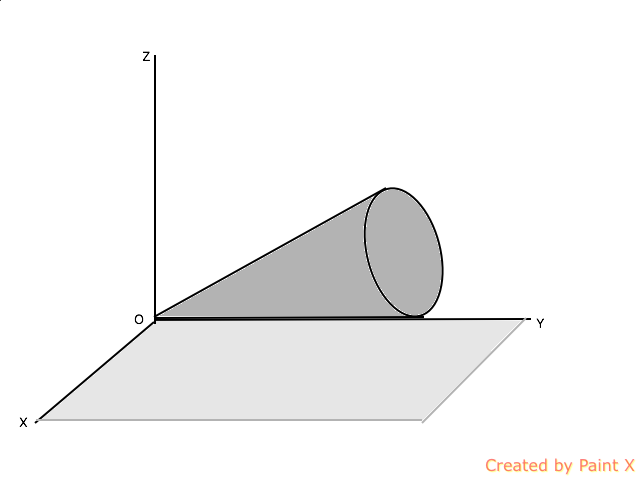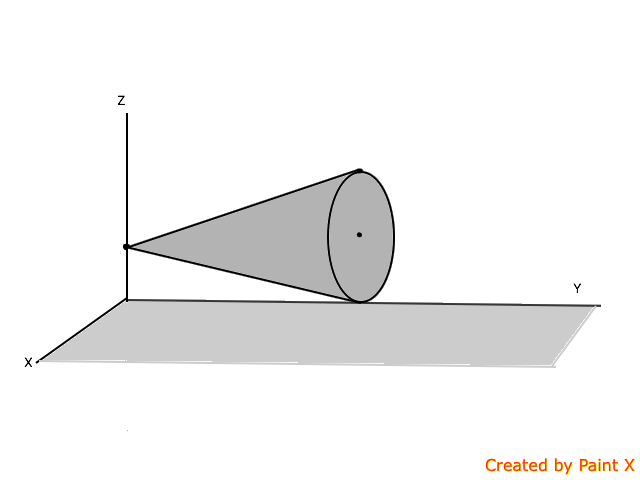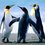# Complex motion part 2

1.A solid cone of length $h$ and half angle $\alpha$ is rolling on a plane about its vortex with an angular velocity of $\Omega\hat{k}$, as shown in Fig. Compute the angular velocity, angular momentum, and kinetic energy of the cone.2.The vertex of the aforementioned cone is fixed on the $z$ axis at a height equal to the radius of the cone. The cone rotates an angular velocity of $\Omega\hat{k}$ about the vertical axis as shown in Fig. Compute the angular velocity, angular momentum, and kinetic energy of the cone.Note by Kudo Shinichi
2 years, 4 months ago

This discussion board is a place to discuss our Daily Challenges and the math and science related to those challenges. Explanations are more than just a solution — they should explain the steps and thinking strategies that you used to obtain the solution. Comments should further the discussion of math and science.

When posting on Brilliant:

• Use the emojis to react to an explanation, whether you're congratulating a job well done , or just really confused .
• Ask specific questions about the challenge or the steps in somebody's explanation. Well-posed questions can add a lot to the discussion, but posting "I don't understand!" doesn't help anyone.
• Try to contribute something new to the discussion, whether it is an extension, generalization or other idea related to the challenge.

MarkdownAppears as
*italics* or _italics_ italics
**bold** or __bold__ bold
- bulleted- list
• bulleted
• list
1. numbered2. list
1. numbered
2. list
Note: you must add a full line of space before and after lists for them to show up correctly
paragraph 1paragraph 2

paragraph 1

paragraph 2

[example link](https://brilliant.org)example link
> This is a quote
This is a quote
    # I indented these lines
# 4 spaces, and now they show
# up as a code block.

print "hello world"
# I indented these lines
# 4 spaces, and now they show
# up as a code block.

print "hello world"
MathAppears as
Remember to wrap math in $$ ... $$ or $ ... $ to ensure proper formatting.
2 \times 3 $2 \times 3$
2^{34} $2^{34}$
a_{i-1} $a_{i-1}$
\frac{2}{3} $\frac{2}{3}$
\sqrt{2} $\sqrt{2}$
\sum_{i=1}^3 $\sum_{i=1}^3$
\sin \theta $\sin \theta$
\boxed{123} $\boxed{123}$

Sort by:

Here is my attempt at the second one. There are two rotations to consider:

1) The rotation of the cone about its own axis
2) The rotation of the cone about the vertical axis

Let $H$ be the height of the cone, $R$ be the radius of the cone, and $\alpha$ be the semi-angle.

$tan \, \alpha = \frac{R}{H} \\ H = \frac{R}{tan \, \alpha}$

Let the angular speed of the cone with respect to the vertical axis be $\omega$, and the angular speed of the cone with respect to its own axis be $\omega'$. They are related as follows (assuming no slipping):

$H \, \omega = R \, \omega'$

Let $I$ and $I'$ be the moments of inertia with respect to the vertical axis and the cone axis, respectively.

$I = \frac{3}{20} \, M \, (R^2 + 4 H^2) \\ I' = \frac{3}{10} \, M \, R^2$

The kinetic energy is then (angular momentum is similar):

$E = \frac{1}{2} I \, \omega^2 + \frac{1}{2} I' \, \omega'^2 \\ \frac{3}{40} \, M \, (R^2 + 4 H^2) \, \omega^2 + \frac{3}{20} \, M \, R^2 \, \omega'^2 \\ = \frac{3}{40} \, M \, R^2 \, \Big( 1 + \frac{4}{tan^2 \, \alpha} \Big) \, \omega^2 + \frac{3}{20} \, M \, R^2 \, \frac{1}{tan^2 \, \alpha} \, \omega^2 \\ = \frac{3}{40} \, M \, R^2 \, \omega^2 + \frac{9}{20} \, M \, R^2 \, \frac{1}{tan^2 \, \alpha} \, \omega^2$

- 2 years, 4 months ago

Thx @Steven Chase sir got.it.(How to.solve first one any ideas ? As such axis of rotation would.be at an angle.from angular.momentum vector right )??

- 2 years, 4 months ago

I'll start thinking about the first one. Did I get the second one right?

- 2 years, 4 months ago

Yes u r right @Steven Chase Sir ( David morin has the first part of the problem in it , but I dont know how they approach the question) . ( they confused me by adding angular momentums )

- 2 years, 4 months ago

Can you post the energy expression for the first part, so I can check my answer when I have it?

- 2 years, 4 months ago

@Steven Chase sir pls share how ur way of solving these 2 problem ? @Aaghaz Mahajan bro pls help u also ?

- 2 years, 4 months ago

Do you know the answers? I think the second one should be fairly easy. The first one will take a bit more work.

- 2 years, 4 months ago

@Steven Chase sir, Can u solve for second part and later first part sir? (Yes I know answers for second part .)

- 2 years, 4 months ago

Ok, I'll do the second part for now

- 2 years, 4 months ago